# Classification using Logistic Regression

In my last article, I explained Linear Regression which is used to predict a continuous value like a stock or a house price. The value can be any real number. But there is another type of important problem in machine learning, the classification problem. This article will cover how logistic regression works and how it can be used to solve the classification problem. If you have not read my last article (https://ahaanpandya.medium.com/linear-regression-explained-868914443188), I highly recommend to read it before this.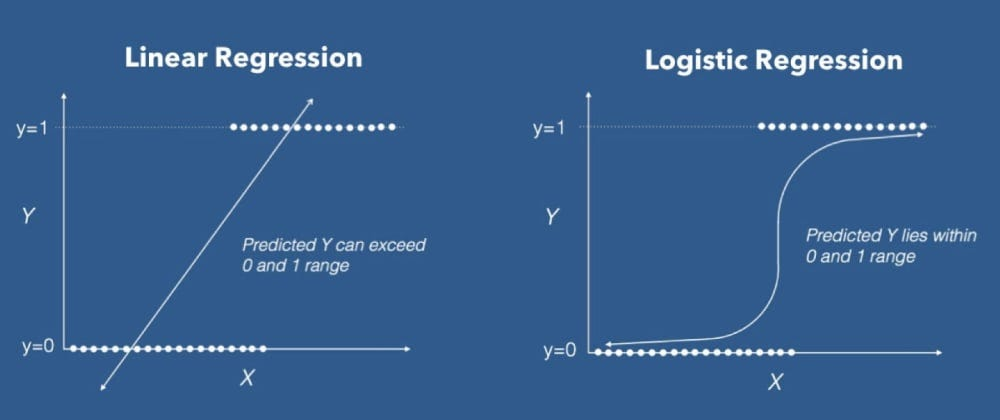The difference between Linear and Logistic Regression, Image from https://dev.to/adityaberi8/logistic-regression-eg1

# Binary Classification

We will first take a look at Binary Classification which involves classifying inputs into only two classes. For example, classifying if a person has a tumour or not, an email is spam or not spam, a customer will buy the product or not, etc. Let’s look at an example where we will try to determine if the customer will purchase a product or not using the gender, age, and salary of the person. Computers work well with numbers so we will use 0 and 1 for the two classes with 0 being the customer did not purchase the product and 1 being the customer did purchase the product. In linear regression, we had a hypothesis function with theta as a parameter which we could use to predict values.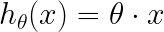This was the hypothesis function for Linear Regression where theta was a vector of parameters

The hypothesis function for logistic regression is similar but with some changes.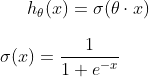This is the hypothesis function where the bottom function is called the sigmoid function or the logistic function

The function we used in this hypothesis function is called the sigmoid/logistic function. This function is very useful because it is a non-linear function that outputs between 0 and 1. The output of our hypothesis function is the probability that our model thinks the x value belongs to the class 1. So if we have a new x value which we want to predict a class for, we plug in the new x value into our hypothesis function. For example, if the hypothesis function outputs 0.3, then the model thinks the x value belongs to the class 1 with a probability of 30% which must mean that the probability it thinks the x value belongs to the class 0 is 70% so the model will predict class 0. If the hypothesis function outputs 0.5 which means the model thinks that the x value is from class 1 with a chance of 50% which also means that it also thinks that it belongs to class 0 with a chance of 50%, then we can predict any of the two classes. The question is, how will we find the parameters theta? We will make a cost function that will measure the error and minimize it using Gradient Descent.

# Cost/Loss Function

The cost function or the loss function will measure the error of our model. The cost function is a very useful metric to know how our model is performing. For Linear Regression, we used the mean squared error cost function but for Logistic regression we will use a different cost function because mean squared error is not so great for a classification problem. Before we see the cost function, let’s quickly recap some machine learning symbols.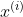This is the i-th training example, this can be only one value or this can be a vector of values if you have multiple features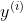This is the answer or the output of i-th training example

We are all set to now see the cost function.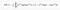This is the cost function for Logistic Regression, Image from https://stats.stackexchange.com/questions/278771/how-is-the-cost-function-from-logistic-regression-derivated

Before you get overwhelmed, let me explain this cost function and why we chose this particular cost function. Let’s say the actual output for a training example is class 1 but our model outputs something closer to 0 like 0.1 which means our model thinks that it is more likely class 0. This cost function will go to infinity as our model output goes closer to 0 which means the model will be heavily penalized by the cost function. This also works the other way around where let’s say the actual output is class 0 but our model outputs closer to 1, then the cost function will again go to infinity which means the model will again be heavily penalized. If the model predicts the right answer then our cost function will go to 0 so the model will be rewarded by the cost function. This is what makes this cost function so good for a classification problem. We can now minimize the cost function using gradient descent. Gradient Descent shouldn’t be new to you if you have read my article on Linear Regression but I will still quickly recap it.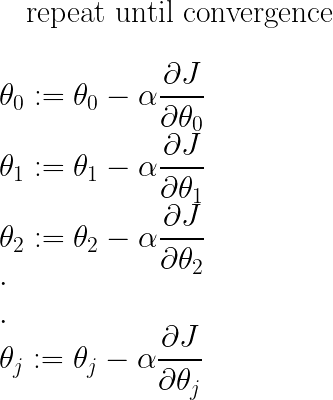This is the gradient descent algorithm where alpha is the learning rate and we update all the parameters theta simultaneously in every iteration of Gradient Descent

# Python Implementation

Now that we know how this algorithm works, we can now use implement in python to solve a classification task. Logistic Regression is a much more complicated algorithm than Linear regression so we will not implement it from scratch. Instead, we will use the Logistic Regression model from the sklearn library. We will predict if a customer will buy a product based on their gender, salary, and age. We will be using the dataset found here : https://www.kaggle.com/dragonheir/logistic-regression

Note- I will be using Pandas and Numpy which are python data science libraries and if you are not comfortable with Numpy and Pandas then you might want to look up a tutorial on those libraries.

`import pandas as pdfrom sklearn.preprocessing import MinMaxScalerfrom sklearn.model_selection import train_test_splitfrom sklearn.linear_model import LogisticRegressiondata_frame = pd.read_csv('/Social_Network_Ads.csv')data_frame.Gender = pd.Categorical(data_frame.Gender).codesX = data_frame[['Gender', 'Age', 'EstimatedSalary']]y = data_frame['Purchased']X = X.to_numpy()y = y.to_numpy()scaler = MinMaxScaler()scaler.fit(X)X = scaler.transform(X)X_train, X_test, y_train, y_test = train_test_split(X, y, test_size=0.2, random_state=42)`

In this piece of code, we first start by reading the data from a csv file and assign the x and y values. The gender column has the words female and male so we replace them by 0 and 1 using pd.Categorical(). We then scale all the x values between 0 and 1 and then split the data into training data and testing data.

`model = LogisticRegression()model.fit(X_train, y_train)def pred(x_value):    x_value = scaler.transform([x_value])    print(model.predict(x_value))`

Next, we make the model and train the model on the training data. We then make our own predict function because we also need to scale the new data we want to predict between 0 and 1 before using the model to predict.

`print(model.score(X_train, y_train))`

We can use this function to print out the accuracy of the model. I got an accuracy of about 90% which is fantastic. This is it for implementing logistic regression in python.

# Multiclass Classification (One vs All Algorithm)

This is going to be a short section explaining multiclass classification because multiclass classification is just essentially binary classification with some changes. In this type of classification you have multiple hypothesis functions. For example, if you have 4 classes, let’s say blue, yellow, green, and red, then you will split it into 4 binary classification problems. You will make one hypothesis function for if our x value is blue or not blue, then you will make a hypothesis function for if your x value is yellow or not, and similarly for all the classes. When you want to predict classes for a new x value, you use all the hypothesis functions and whichever one has the highest value, you return that class. The python implementation for multiclass classification is identical as the binary classification so I won’t be showing that here.

# Conclusion

Logistic Regression is one of the most widely used classification algorithms today. It can also be implemented fast using python. I hope you liked this article and learnt something new.